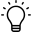# How to calculate projection in cmaHow to calculate projection in cma

There is an option of "auto calculate in" the software for projection calculation, you have to check mark on it.If you want to check growth percentage wise then you can also enter percentage ,the software calculates the forward projection in that ratio.Hope this Helps